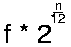Perception of Sound

 The human ear is sensitive to both the volume and frequency of sounds.

 Humans can hear frequencies from 20Hz to 20000Hz.

 Tones which have frequencies which are related by small rational ratios are perceived as being related.

 In music, tones which have frequencies which are a power of two apart are considered to be the same note in different octaves.

 In music, tones which have frequencies which are a power of two apart are considered to be the same note in different octaves.

 Each octave is split into 12 notes which are geometrically spaced within the octave. Given a musical note of frequency f, the frequency of the nth note above f is given by:This equation works for both positive an negative values on n. With n positive, you get notes above the starting note and for n negative we get notes below.